# Array Manipulation

Use the new array manipulation functions in Excel 365 to quickly combine, shape and resize arrays.

### VSTACK

The VSTACK function in Excel 365 vertically stacks multiple arrays into a single array.Note: the VSTACK function, entered into cell B5, fills multiple cells. This behavior is called spilling.

### HSTACK

The HSTACK function in Excel 365 horizontally stacks multiple arrays into a single array.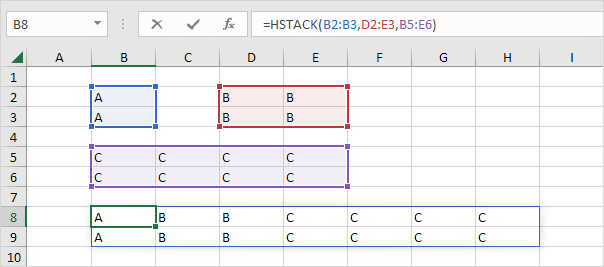### TOROW

The TOROW function in Excel 365 converts a 2D array into a single row.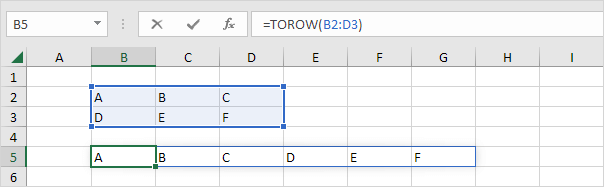### TOCOL

The TOCOL function in Excel 365 converts a 2D array into a single column.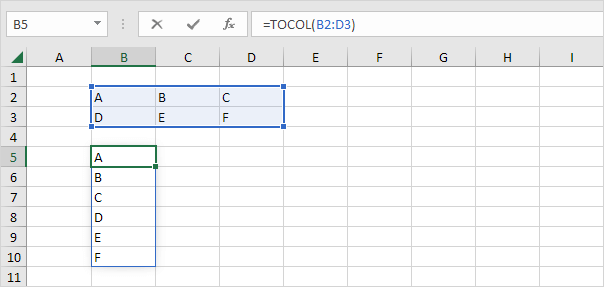### WRAPROWS

The WRAPROWS function converts a 1D array into a 2D array. WRAPROWS starts a new row after a specified number of elements (second argument).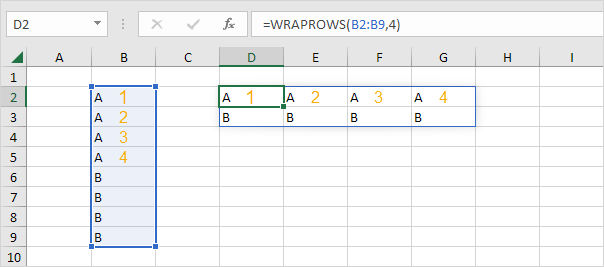### WRAPCOLS

The WRAPCOLS function converts a 1D array into a 2D array. WRAPCOLS starts a new column after a specified number of elements (second argument).### TAKE

The TAKE function extracts a subset of an array. You can start in each corner by using positive and negative arguments.

1. The TAKE function below extracts a 4 by 2 array (starting in the upper-left corner).2. The TAKE function below extracts a 3 by 1 array (starting in the bottom-right corner).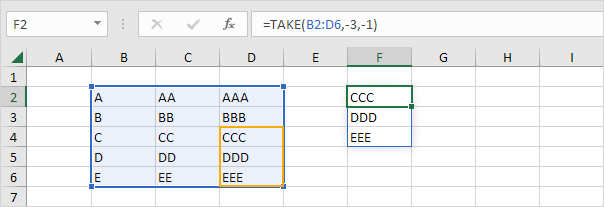### CHOOSEROWS

The CHOOSEROWS function in Excel 365 returns specific rows from an array. The CHOOSEROWS function below returns the 3rd and the 5th row from an array.Note: use CHOOSECOLS to return specific columns from an array. For example, download the Excel file and try to return the 2nd column from this array. Practice makes perfect!

Go to Next Chapter: Sort